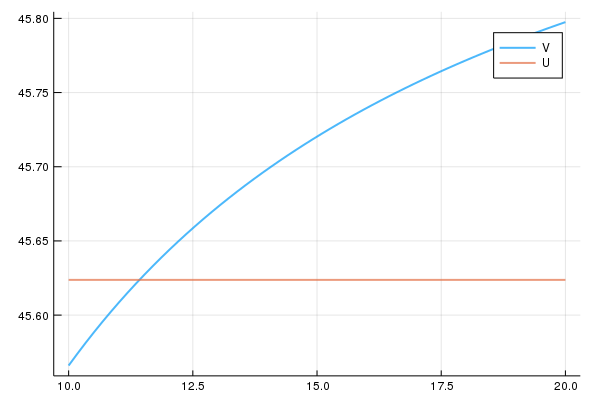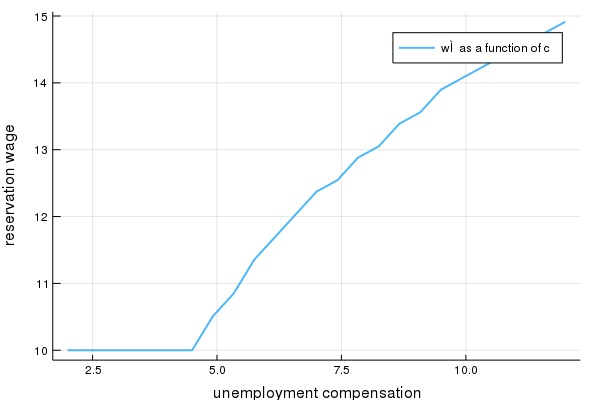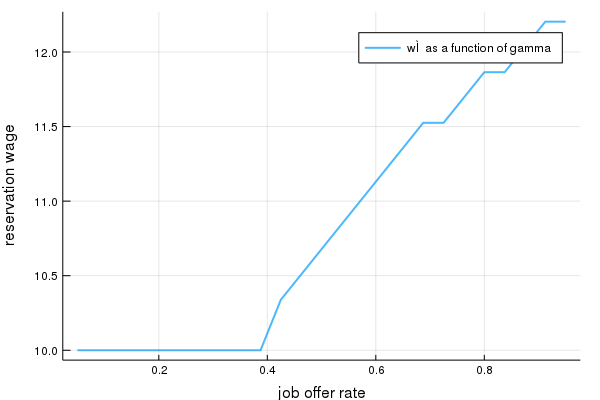We are working to support a site-wide PDF but it is not yet available. You can download PDFs for individual lectures through the download badge on each lecture page.How to read this lecture...

Code should execute sequentially if run in a Jupyter notebook

• See the set up page to install Jupyter, Julia (1.0+) and all necessary libraries
• Please direct feedback to contact@quantecon.org or the discourse forum
• For some notebooks, enable content with "Trust" on the command tab of Jupyter lab
• If using QuantEcon lectures for the first time on a computer, execute ] add InstantiateFromURL inside of a notebook or the REPL

# Job Search II: Search and Separation¶

## Overview¶

Previously we looked at the McCall job search model [McC70] as a way of understanding unemployment and worker decisions

One unrealistic feature of the model is that every job is permanent

In this lecture we extend the McCall model by introducing job separation

Once separation enters the picture, the agent comes to view

• the loss of a job as a capital loss, and
• a spell of unemployment as an investment in searching for an acceptable job

### Setup¶

In :
using InstantiateFromURL
activate_github("QuantEcon/QuantEconLecturePackages", tag = "v0.9.7");

In :
using LinearAlgebra, Statistics, Compat
using Distributions, Expectations, Parameters, NLsolve, Plots


## The Model¶

The model concerns the life of an infinitely lived worker and

• the opportunities he or she (let’s say he to save one character) has to work at different wages
• exogenous events that destroy his current job
• his decision making process while unemployed

The worker can be in one of two states: employed or unemployed

He wants to maximize

$${\mathbb E} \sum_{t=0}^\infty \beta^t u(Y_t) \tag{1}$$

The only difference from the baseline model is that we’ve added some flexibility over preferences by introducing a utility function $u$

It satisfies $u'> 0$ and $u'' < 0$

### Timing and Decisions¶

Here’s what happens at the start of a given period in our model with search and separation

If currently employed, the worker consumes his wage $w$, receiving utility $u(w)$

If currently unemployed, he

• receives and consumes unemployment compensation $c$
• receives an offer to start work next period at a wage $w'$ drawn from a known distribution $p_1, \ldots, p_n$

He can either accept or reject the offer

If he accepts the offer, he enters next period employed with wage $w'$

If he rejects the offer, he enters next period unemployed

When employed, the agent faces a constant probability $\alpha$ of becoming unemployed at the end of the period

(Note: we do not allow for job search while employed—this topic is taken up in a later lecture)

## Solving the Model using Dynamic Programming¶

Let

• $V(w)$ be the total lifetime value accruing to a worker who enters the current period employed with wage $w$
• $U$ be the total lifetime value accruing to a worker who is unemployed this period

Here value means the value of the objective function (1) when the worker makes optimal decisions at all future points in time

Suppose for now that the worker can calculate the function $V$ and the constant $U$ and use them in his decision making

Then $V$ and $U$ should satisfy

$$V(w) = u(w) + \beta [(1-\alpha)V(w) + \alpha U ] \tag{2}$$

and

$$U = u(c) + \beta \sum_i \max \left\{ U, V(w_i) \right\} p_i \tag{3}$$

Let’s interpret these two equations in light of the fact that today’s tomorrow is tomorrow’s today

• The left hand sides of equations (2) and (3) are the values of a worker in a particular situation today
• The right hand sides of the equations are the discounted (by $\beta$) expected values of the possible situations that worker can be in tomorrow
• But tomorrow the worker can be in only one of the situations whose values today are on the left sides of our two equations

Equation (3) incorporates the fact that a currently unemployed worker will maximize his own welfare

In particular, if his next period wage offer is $w'$, he will choose to remain unemployed unless $U < V(w')$

Equations (2) and (3) are the Bellman equations for this model

Equations (2) and (3) provide enough information to solve out for both $V$ and $U$

Before discussing this, however, let’s make a small extension to the model

### Stochastic Offers¶

Let’s suppose now that unemployed workers don’t always receive job offers

Instead, let’s suppose that unemployed workers only receive an offer with probability $\gamma$

If our worker does receive an offer, the wage offer is drawn from $p$ as before

He either accepts or rejects the offer

Otherwise the model is the same

With some thought, you will be able to convince yourself that $V$ and $U$ should now satisfy

$$V(w) = u(w) + \beta [(1-\alpha)V(w) + \alpha U ] \tag{4}$$

and

$$U = u(c) + \beta (1 - \gamma) U + \beta \gamma \sum_i \max \left\{ U, V(w_i) \right\} p_i \tag{5}$$

### Solving the Bellman Equations¶

We’ll use the same iterative approach to solving the Bellman equations that we adopted in the first job search lecture

Here this amounts to

1. make guesses for $U$ and $V$
2. plug these guesses into the right hand sides of (4) and (5)
3. update the left hand sides from this rule and then repeat

In other words, we are iterating using the rules

$$V_{n+1} (w_i) = u(w_i) + \beta [(1-\alpha)V_n (w_i) + \alpha U_n ] \tag{6}$$

and

$$U_{n+1} = u(c) + \beta (1 - \gamma) U_n + \beta \gamma \sum_i \max \{ U_n, V_n(w_i) \} p_i \tag{7}$$

starting from some initial conditions $U_0, V_0$

Formally, we can define a “Bellman operator” T which maps:

$$TV(\cdot) = u(\cdot) + \beta (1-\alpha)V(\cdot) + \alpha U \tag{8}$$

In which case we are searching for a fixed point

$$TV^{*} = V^* \tag{9}$$

As before, the system always converges to the true solutions—in this case, the $V$ and $U$ that solve (4) and (5)

A proof can be obtained via the Banach contraction mapping theorem

## Implementation¶

Let’s implement this iterative process

In :
using Distributions, LinearAlgebra, Compat, Expectations, Parameters, NLsolve, Plots

function solve_mccall_model(mcm; U_iv = 1.0, V_iv = ones(length(mcm.w)), tol = 1e-5,
iter = 2_000)
# α, β, σ, c, γ, w = mcm.α, mcm.β, mcm.σ, mcm.c, mcm.γ, mcm.w
@unpack α, β, σ, c, γ, w, dist, u = mcm

# parameter validation
@assert c > 0.0
@assert minimum(w) > 0.0 # perhaps not strictly necessary, but useful here

# necessary objects
u_w = u.(w, σ)
u_c = u(c, σ)
E = expectation(dist) # expectation operator for wage distribution

# Bellman operator T. Fixed point is x* s.t. T(x*) = x*
function T(x)
V = x[1:end-1]
U = x[end]
[u_w + β * ((1 - α) * V .+ α * U); u_c + β * (1 - γ) * U + β * γ * E * max.(U, V)]
end

# value function iteration
x_iv = [V_iv; U_iv] # initial x val
xstar = fixedpoint(T, x_iv, iterations = iter, xtol = tol).zero
V = xstar[1:end-1]
U = xstar[end]

# compute the reservation wage
wbarindex = searchsortedfirst(V .- U, 0.0)
if wbarindex >= length(w) # if this is true, you never want to accept
w̄ = Inf
else
w̄ = w[wbarindex] # otherwise, return the number
end

# return a NamedTuple, so we can select values by name
return (V = V, U = U, w̄ = w̄)
end

Out:
solve_mccall_model (generic function with 1 method)

The approach is to iterate until successive iterates are closer together than some small tolerance level

We then return the current iterate as an approximate solution

Let’s plot the approximate solutions $U$ and $V$ to see what they look like

We’ll use the default parameterizations found in the code above

In :
# a default utility function
u(c, σ) = (c^(1 - σ) - 1) / (1 - σ)

# model constructor
McCallModel = @with_kw (α = 0.2,
β = 0.98, # discount rate
γ = 0.7,
c = 6.0, # unemployment compensation
σ = 2.0,
u = u, # utility function
w = range(10, 20, length = 60), # wage values
dist = BetaBinomial(59, 600, 400)) # distribution over wage values

Out:
#5 (generic function with 2 methods)
In :
# plots setting
gr(fmt=:png);

mcm = McCallModel()
@unpack V, U = solve_mccall_model(mcm)
U_vec = fill(U, length(mcm.w))

plot(mcm.w, [V U_vec], lw = 2, α = 0.7, label = ["V" "U"])

Out:The value $V$ is increasing because higher $w$ generates a higher wage flow conditional on staying employed

At this point, it’s natural to ask how the model would respond if we perturbed the parameters

These calculations, called comparative statics, are performed in the next section

## The Reservation Wage¶

Once $V$ and $U$ are known, the agent can use them to make decisions in the face of a given wage offer

If $V(w) > U$, then working at wage $w$ is preferred to unemployment

If $V(w) < U$, then remaining unemployed will generate greater lifetime value

Suppose in particular that $V$ crosses $U$ (as it does in the preceding figure)

Then, since $V$ is increasing, there is a unique smallest $w$ in the set of possible wages such that $V(w) \geq U$

We denote this wage $\bar w$ and call it the reservation wage

Optimal behavior for the worker is characterized by $\bar w$

• if the wage offer $w$ in hand is greater than or equal to $\bar w$, then the worker accepts
• if the wage offer $w$ in hand is less than $\bar w$, then the worker rejects

If $V(w) < U$ for all $w$, then the function returns np.inf

Let’s use it to look at how the reservation wage varies with parameters

In each instance below we’ll show you a figure and then ask you to reproduce it in the exercises

### The Reservation Wage and Unemployment Compensation¶

First, let’s look at how $\bar w$ varies with unemployment compensation

In the figure below, we use the default parameters in the McCallModel tuple, apart from c (which takes the values given on the horizontal axis)As expected, higher unemployment compensation causes the worker to hold out for higher wages

In effect, the cost of continuing job search is reduced

### The Reservation Wage and Discounting¶

Next let’s investigate how $\bar w$ varies with the discount rate

The next figure plots the reservation wage associated with different values of $\beta$Again, the results are intuitive: More patient workers will hold out for higher wages

### The Reservation Wage and Job Destruction¶

Finally, let’s look at how $\bar w$ varies with the job separation rate $\alpha$

Higher $\alpha$ translates to a greater chance that a worker will face termination in each period once employedOnce more, the results are in line with our intuition

If the separation rate is high, then the benefit of holding out for a higher wage falls

Hence the reservation wage is lower

## Exercises¶

### Exercise 1¶

Reproduce all the reservation wage figures shown above

### Exercise 2¶

Plot the reservation wage against the job offer rate $\gamma$

Use

In :
γ_vals = range(0.05,  0.95, length = 25)

Out:
0.05:0.0375:0.95

## Solutions¶

### Exercise 1¶

Using the solve_mccall_model function mentioned earlier in the lecture, we can create an array for reservation wages for different values of $c$, $\beta$ and $\alpha$ and plot the results like so

In :
c_vals = range(2,  12, length = 25)

models = [McCallModel(c = cval) for cval in c_vals]
sols = solve_mccall_model.(models)
w̄_vals = [sol.w̄ for sol in sols]

plot(c_vals,
w̄_vals,
lw = 2,
α = 0.7,
xlabel = "unemployment compensation",
ylabel = "reservation wage",
label = "w̄ as a function of c")

Out:Note that we could’ve done the above in one pass (which would be important if, for example, the parameter space was quite large)

In :
w̄_vals = [solve_mccall_model(McCallModel(c = cval)).w̄ for cval in c_vals];
# doesn't allocate new arrays for models and solutions


### Exercise 2¶

Similar to above, we can plot $\bar w$ against $\gamma$ as follows

In :
γ_vals = range(0.05,  0.95, length = 25)

models = [McCallModel(γ = γval) for γval in γ_vals]
sols = solve_mccall_model.(models)
w̄_vals = [sol.w̄ for sol in sols]

plot(γ_vals, w̄_vals, lw = 2, α = 0.7, xlabel = "job offer rate",
ylabel = "reservation wage", label = "w̄ as a function of gamma")

Out:As expected, the reservation wage increases in $\gamma$

This is because higher $\gamma$ translates to a more favorable job search environment

Hence workers are less willing to accept lower offers

• Share page